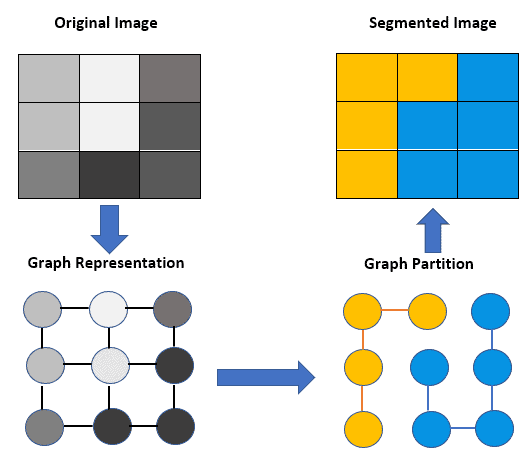## 1. Introduction

Image processing is essential for computer vision since it involves analyzing, understanding, and manipulating images. Furthermore, image segmentation is a crucial task in image processing. It involves dividing an image into several meaningful regions or segments based on some properties, such as color, texture, and brightness.

In this article, we’ll study the concept of Graph-Based Segmentation (GBS), how it works, and its various applications.

## 2. Graph-based Segmentation

GBS involves the application of a graph theory to construct a representation of an image in the form of a graph. In this approach, each image pixel is represented as a node, while the edges connecting the nodes represent the degree of similarity between the corresponding pixels.

The main objective of GBS is to divide an image into separate regions, each one representing a segment in the image.

Moreover, GBS uses graph partitioning algorithms aiming to reduce the cost of separating segments in the image by minimizing the total weight of the edges that need to be cut.

## 3. How Does Graph-Based Segmentation Work?

GBS algorithms follow a set of standard steps for segmenting images. Hence, this section outlines the steps involved in it.

In the first step, we construct a graph to represent the image, where each pixel is a node. So, we must define the weights of the edges between the nodes. It bases on the similarity or dissimilarity between the pixels, like the difference in color or intensity values.

Then, we partition the graph into disjoint regions employing a graph partitioning algorithm. This algorithm’s goal is to minimize the weights of the edges between the segments.

Finally, we refine the segments by merging or splitting them based on several criteria, such as size, shape, or texture.

The figure next shows the steps of the GBS and how an image is transformed into a segmented image:## 4. Graph Partitioning Algorithms

This section will introduce three different graph partitioning algorithms: spectral clustering, normalized cut, and minimum cut.

It’s relevant to highlight that graph partitioning has a critical role in GBS. It ensures that the resulting segments are meaningful and accurate.

### 4.1. Spectral Clustering

Spectral clustering is a popular algorithm for GBS. It’s based on the eigenvectors of the Laplacian matrix, which captures the connectivity of the graph.

Spectral clustering works by first computing the Laplacian matrix of the graph. Thus, it finds the eigenvectors corresponding to the smallest eigenvalues. Finally, it applies k-means clustering to the eigenvectors to obtain the segments.

### 4.2. Normalized Cut

The normalized cut is another algorithm used for graph partitioning. It bases on the notion of minimizing the ratio of the cut cost to the sum of the weights of the edges within each segment.

The normalized cut algorithm recursively divides the graph into smaller sub-graphs. It occurs until the sub-graphs meet predefined stopping criteria.

### 4.3. Minimum Cut

Minimum cut is a classic algorithm for performing the graph partitioning task. It works by finding the minimum cut in a graph, which means the cut with the lowest weight.

The minimum-cut algorithm partitions the graph into two segments by removing the edges with the smallest weights until it’s disconnected.

Overall, GBS is a powerful and flexible method that may produce accurate and precise segmentation. However, it requires careful parameter tuning and can be computationally expensive.

The table shown below provides a comparison between the advantages and disadvantages of the GBS:## 6. Applications of Graph-Based Segmentation

GBS has numerous applications across various industries. In this section, we’ll discuss some of them.

First, object recognition and tracking in real-time video streams application use GBS algorithms. By segmenting objects, these systems can identify and track them over time, enabling the development of autonomous vehicles and surveillance systems.

GBS is a popular method in medical imaging to segment and analyze images of organs, tissues, and cells since it can help diagnose diseases and conditions and assist in surgical planning. For example, we can use it to detect and segment tumors in medical images.

Also, autonomous driving systems use GBS to detect and segment objects in the environment, such as pedestrians, vehicles, and traffic signs.

Furthermore, GBS is used in image editing and manipulating applications, like image segmentation and superpixel generation, since it can help separate the foreground and background of an image, making it easier to apply effects and filters.

Robotics and automation application use GBS to detect objects from images and identify them based on their characteristics. It’s particularly beneficial in industries where object detection and manipulation are necessary.

In summary, the GBS’s flexibility, accuracy, and speed make it a valuable tool in various applications.

## 7. Conclusion

In conclusion, graph-based segmentation is a powerful and flexible technique for image processing, presenting multiple applications in different areas.

GBS can produce accurate and precise results by considering local and global information in an image. But, to do that, GBS requires precise parameter tuning and can be computationally expensive.